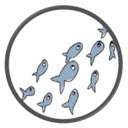Free editor online | DOC > | XLS > | PPT ># Guzinta Math: Linear Equations in Chrome with OffiDocs## DESCRIPTION

For one-on-one or whole-class mathematics instruction, use this Guzinta Math lesson as a supplement for teaching building linear equations, using inverse operations to simplify expressions and solve equations of the form px + q = r, and circle circumference and area problems--all key concepts in 7th grade.

NEW 5.0 version contains a slicker look, an exploratory module on graphs of linear equations and points using an interactive graphing tool, and extra practice questions which appear progressively over 3 weeks from starting the app.

This lesson app also includes the popular Practice Meter feature, which can keep track of each student's practice level for this app across sessions.

The purpose of the Practice Meter is to motivate students to come back and revisit this content and complete modules at regular intervals.

Revisit the lesson app to keep your Practice Meter out of the red (or in the blue).

It becomes easier over time to maintain your practice level above the key levels of 80 or 25, because the meter depletes much more slowly after a week, then a month, then 3 months, has passed.

The math lesson app also includes a NOTES feature: helpful tips for students, self-explanation questions, further information, and even suggestions for extension or family involvement--for every question and example! Guzinta Math: Linear Equations focuses on representing relationships given in situations as equations of the form px + q = r, or y = mx + b.

The relationships highlighted here are from a variety of real-world situations and pure mathematical forms.

Students also use circumference and area formulas to solve problems involving circles.

For one-on-one or whole-class mathematics instruction, use this Guzinta Math lesson as a supplement for teaching building linear equations and circle circumference and area problems--all key concepts in 7th grade.

The app includes detailed instructor notes for each guided practice problem, with notes about extending discussion around each worked example and problem.

Instructors and/or students can turn on and use the new canvas feature (with improved writing functionality) in the app to write all over the screen--take notes, highlight key terms and ideas, show work, etc.